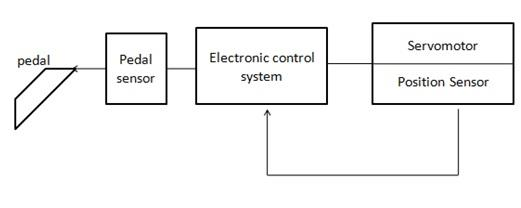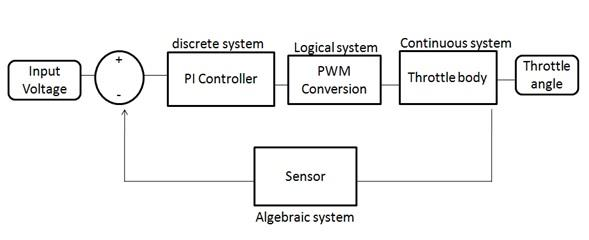### Simulink in MATLAB, 3D Animation,Code Inspector - MATLAB Assignment Help

-  Simulink is a visual design tool in the MATLAB that facilitates Model Based Design.

- Simulink provides graphical modeling environment for design and development of discrete, continuous and hybrid systems.

- Simulink provides a convenient simulation environment where the developed algorithm which is developed after deriving certain mathematical formula can be simulated for the stipulated simulation period (start time - 0 to stop time can be any positive integer which is set in the configuration parameters) and output results for each block or the outputs can be logged for each time steps and can be used for analysis, refinement and validation of design.

- Simulink provides immediate access to the various ranges of tools for algorithm development, data visualization, data analysis and numerical computation.

- We can use Simulink to visually develop the following

• Physical system plants (system differential equations )-continuous systems
• controllers/control algorithms(control difference equations)-discrete systems
• filters and sensors

- It is a complete environment for model the system, simulate it and implementing dynamic and embedded systems.

- It is used for designing and tests the linear, non linear, discrete-time, continuous time, hybrid and multi rate systems

- It is used for applications in controls, DSP, communications and system engineering

- It is open architecture allows integration of models from other environments.

- Model Based Design with Simulink is the process of using the Simulink models starting from the requirements phase to product deployment in the product development.

Stage 1:  executable requirements in models are unambiguous

• illustrates requirements in models are unambiguous

Stage 2:  Design with Simulink models

• Virtual prototype models are complete, flexible and inexpensive

Stage 3:  Implementation by using Auto code generation

• Automatic code generation eliminates inconsistency and coding error

Stage 4:  Continuous test and verification of developed system

• Testing hardware allows early error detection.

To open Simulink type in the MATLAB workspace

Or click on Simulink library button in Mat lab header and then click on new button on the library browser toolbar.

- Design of electronic throttle control system which replaces the mechanical linkage between accelerator pedal and throttle.

Here in electronic throttle control system where pedal input is passed to pedal position sensor and then an electronic control system is present and there actual throttle body which consists of servomotor which is used to change the throttle plate and a sensor is present to detect the position of throttle which is again given back to the electronic systemSo the whole electronic throttle system can be divided into the different subsystems as the above

1.  PI controller

Where the difference between the actual throttle plate position and the desired throttle plate position is fed to the PI Controller

PI controller is the discrete system. Discrete system can be represented by using the difference equations.

y(k) = yp(k)+yi(k)

yp(k) = kpe(k)

yi(k) = yi(k-1)+kie(k)

kp = proportional gain

ki = integration gain

ek = error

yp(k),yi(k)-- discrete states

By using the Simulink by using discrete blocks in the Simulink library in the Simulink like delay blocks we can design that.

2.  PWM Conversion:

• Here the controller output is converted to the duty and direction
•  Here based on the logical equations we can design those using math operations blocks and logical blocks in the Simulink.

3.  Throttle Body:

• Here throttle body (continuous system) is derived using the differential   equations
• Here we can use the continuous block like integrals blocks in the Simulink.

4.  sensor:

Here the sensors is the potentiometer(algebraic system) where the input is the throttle position from the throttle body and convert to the voltage where it will give the actual throttle plate position is converted into the voltage and it is given to the difference block where the actual pedal voltage- desired pedal voltage is feed again to the controller.

Here the whole systems which contains all the discrete and continuous systems (hybrid systems) with difference and differential, algebraic equations we can model the whole system.

We can simulate and formalize the requirements in the unambiguous way

We can eliminate the coding errors like syntax issues and formatting issue by using the auto code generation tools like Real Time Workshop or embedded coder

We can test easily by harnessing the models using MIL, SIL, PIL by using the simulated host environment itself

Cost of the hardware testing is reduced a lot by using the matlab tools like scape goat by simulating Io and dumping code in the actual target.

By using the Simulink we can test rigorously at each phase so the cost of poor quality reduced.

Even though the issues in the validation phase can also be fixed with less number of  efforts by using small altercations using the Simulink library.

Cost of maintenance of the software will be very easy using the model based design

- Dynamic position of ships in heavy seas with simulations using the scale models in laboratory.

- Next generation network audio products by simulating signals transmitted over a network.

- Improve the race car performance by designing a system for the automatic testing of suspension systems.

- Teach the computer programming to undergraduates by developing a test and measurement laboratory that poses authentic engineering problems to the students.

- Use help for any block related queries for better understanding of blocks in Simulink

- Use design verifier to know whether there are any problems in your design.

- Use Simulink Model Advisor to fix or propose some solutions for the fixes in your design.

Choose the model or subsystem that you like to run checks on click OK

Available checks:

Performance: Checks under Code generation efficiency

Accuracy: Simulation runtime accuracy

Predictability: Managing Library links and Variant checks

Consistency: Requirement consistency checking

Adherence to standards: Modeling standards for MAAB

- For m scripting u can use line by line debugging or evaluating selected sections and also by using break points.

We offer Simulink assignment help, Simulink assignment writing help, assessments writing service, Simulink tutors support, step by step solutions to Simulink problems, Simulink answers, MATLAB assignment experts help online. Our MATLAB assignment help service is most popular and browsed all over the world for each grade level.

There are key services in Simulink which are listed below:-

• Assignment Help
• Homework Help
• Simulink in MATLAB Assessments Writing Service
• Solutions to problems
• MATLAB papers writing and editing
• Paper formatting and referencing
• Simulink MATLAB research papers writing help
• Thesis and dissertation help
• Experts support 24x7
• Online tutoring

Why choose us - The first thing come in your mind that why choose us why not others what is special and different about us in comparison to other site. As we told you our team of expert, they are best in their field and we are always live to help you in your assignment which makes it special.

Key features of services are listed below:

• Confidentiality of student private information
• 100% unique and original solutions
• Step by step explanations of problems
• Minimum 4 minutes turnaround time - fast and reliable service
• Secure payment options
• On time delivery
• Unlimited clarification till you are done
• Guaranteed satisfaction
• Affordable price to cover maximum number of students in service
• Easy and powerful interface to track your orderMost Matched Writing Services:-Get Academic Excellence with Best Skilled Tutor! Order Assignment Now!